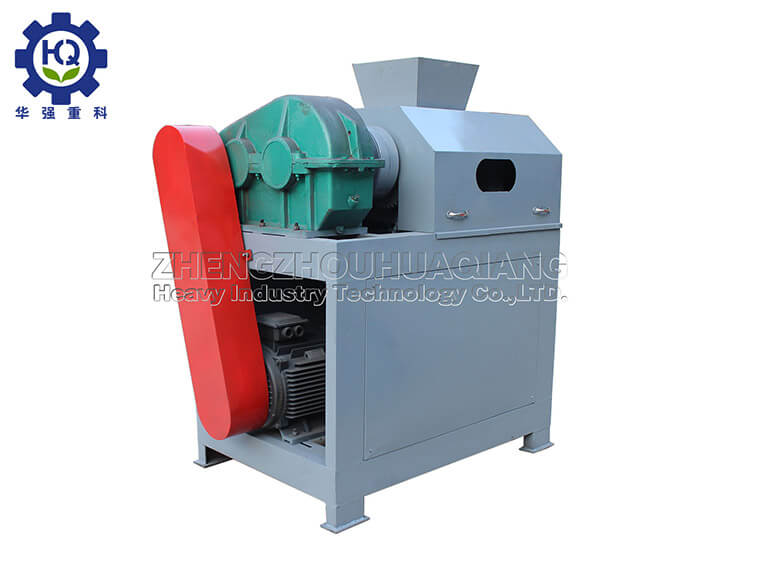Blog

# Quick Calculation Method of Finely Solving Fertilizer Application

2022/06/30

## Quick calculation of fertilizer application rate

The nutrient balance method is mainly used to determine the amount of fertilizer. The basic concept of nutrient balance method is that the nutrient absorption of crops is equal to the sum of the nutrient supply of soil and fertilizer.

Organic fertilizer making machine converts manure and straw into organic fertilizer. Part of the nutrients that fertilizer provides to crops must be carried out through fertilization. However, the amount of fertilizer applied to crops is not exactly the same as the nutrient supply of fertilizers. Because only part of the nutrients put into the farmland is absorbed and utilized by the crops of the current season, considering the fertilizer utilization factor, the amount of fertilizer can be calculated by the following formula:
Planned crop fertilizer amount (kg) = (total amount of nutrients required for planned yield - soil nutrient supply) × fertilizer nutrient content × fertilizer utilization rate (%)
The amount of fertilizer applied to crops refers to the amount of a certain nutrient element. For specific chemical fertilizer varieties, the actual amount of chemical fertilizer should be calculated by the following formula:
Planned crop fertilizer amount (kg) = physical fertilizer amount (kg) × active ingredient content (%)## Among them:

1. The total amount of nutrients required for the planned output (kg) = (planned output/100) × the number of nutrients required for each 100kg output.
The planned output is to increase the average output of local crops by 10% to 15% in three years.

2. Soil nutrient supply (kg)=(fertilizer-free area yield/100)×the number of nutrients required for each 100kg yield.
The amount of soil fertility is generally estimated by soil sampling and testing. In the absence of laboratory conditions, it can also be estimated by the yield without fertilization (blank yield).

3. In general, the current season utilization rate of chemical fertilizers is: nitrogen fertilizer 30%-35%, phosphate fertilizer 20%-25%, and potash fertilizer 25%-35%. According to this ratio, npk fertilizer can be processed with the double roller granulator.